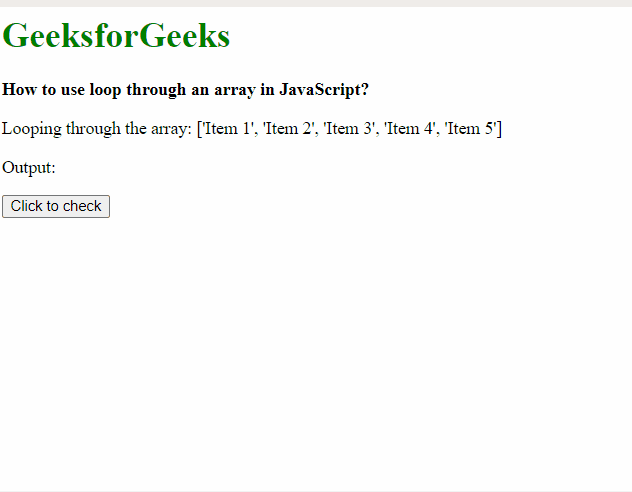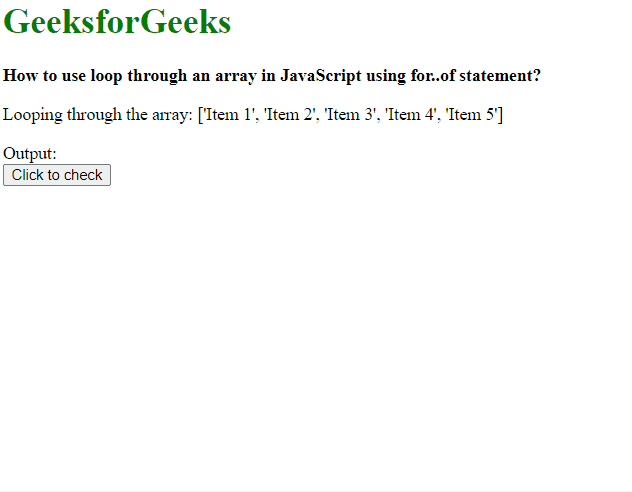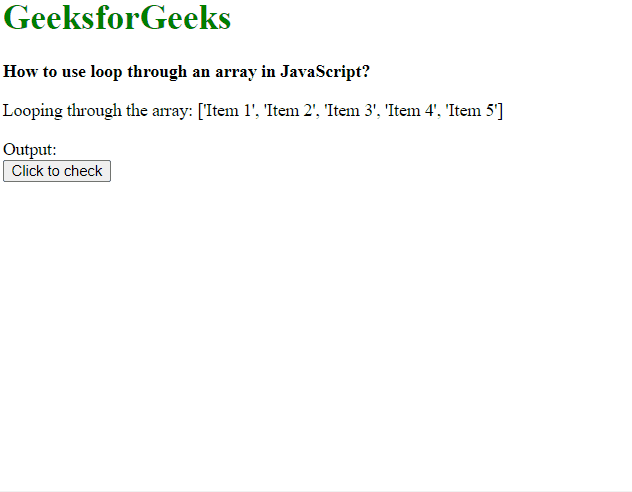Open in App
Not now

# How to use loop through an array in JavaScript ?

• Last Updated : 21 Dec, 2022

In this article, we will see how to use the various type of loops available in Javascript with Javascript arrays. We will see the implementation of forEach() loop, for..of loop and for loop in this article.

Method 1: Using the forEach() method.

The forEach() method is used to execute code for each element in the array by looping through each of them.

Syntax:

```array.forEach( arrayElement => {
// lines of code to execute
});```

Example: In this example, we will loop through an array using the forEach() method and print the values.

## html

 `<``h1` `style``=``"color: green"``>``    ``GeeksforGeeks```` ` `<``b``>``    ``How to use loop through an``    ``array in JavaScript?```` ` `<``p``>``    ``Looping through the array: ['Item 1',``    ``'Item 2', 'Item 3', 'Item 4', 'Item 5']```` ` `<``p``>Output:``<``div` `class``=``"output"``>```` ` `<``button` `onclick``=``"loopArray()"``>``    ``Click to check```` ` `<``script` `type``=``"text/javascript"``>``    ``function loopArray() {``        ``list = ['Item 1', 'Item 2', 'Item 3',``                ``'Item 4', 'Item 5'];``         ` `        ``list.forEach(element => {``            ``document.querySelector('.output').innerHTML``                    ``+= '<``li``>' + element + '';``        ``});``    ``}```

Output:Method 2: Using the for…of statement.

The for…of statement can be used to loop through iterable objects and perform the required functions. Iterable objects include arrays, strings, and other array-like objects.

Syntax:

```for (arrayElement of array) {
// lines of code to execute
}```

Example: In this example, we will loop through an array using the for..of statement and print the values.

## html

 `<``h1` `style``=``"color: green"``>``    ``GeeksforGeeks```` ` `<``b``>``    ``How to use loop through an``    ``array in JavaScript using for..of statement?```` ` `<``p``>``    ``Looping through the array: ['Item 1',``    ``'Item 2', 'Item 3', 'Item 4', 'Item 5']```` ` `<``div``>Output:``    ``<``div` `class``=``"output"``>```` ` `<``button` `onclick``=``"loopArray()"``>``    ``Click to check```` ` `<``script` `type``=``"text/javascript"``>``    ``function loopArray() {``        ``list = ['Item 1', 'Item 2', 'Item 3',``                ``'Item 4', 'Item 5'];``         ` `        ``for (element of list) {``            ``document.querySelector('.output').innerHTML``                ``+= '<``li``>' + element + '';``        ``}``    ``}```

Output:Method 3: Using the basic for loop.

The default for loop can be used to iterate through the array and each element can be accessed by its respective index. Syntax:

```for (i = 0; i < list.length; i++) {
// lines of code to execute
}```

Example: In this example, we will loop through an array using the for loop and print the values.

## html

 `<``h1` `style``=``"color: green"``>``    ``GeeksforGeeks```` ` `<``b``>``    ``How to use loop through an``    ``array in JavaScript?```` ` `<``p``>``    ``Looping through the array: ['Item 1',``    ``'Item 2', 'Item 3', 'Item 4', 'Item 5']```` ` `<``div``>Output:``    ``<``div` `class``=``"output"``>```` ` `<``button` `onclick``=``"loopArray()"``>``    ``Click to check```` ` `<``script` `type``=``"text/javascript"``>``    ``function loopArray() {``        ``list = ['Item 1', 'Item 2', 'Item 3',``                ``'Item 4', 'Item 5'];``         ` `        ``for (i = 0; i < ``list.length``; i++) {``            ``document.querySelector('.output').innerHTML``                    ``+= '
• ' + list[i] + '';``        ``}``    ``}```
• Output:My Personal Notes arrow_drop_up